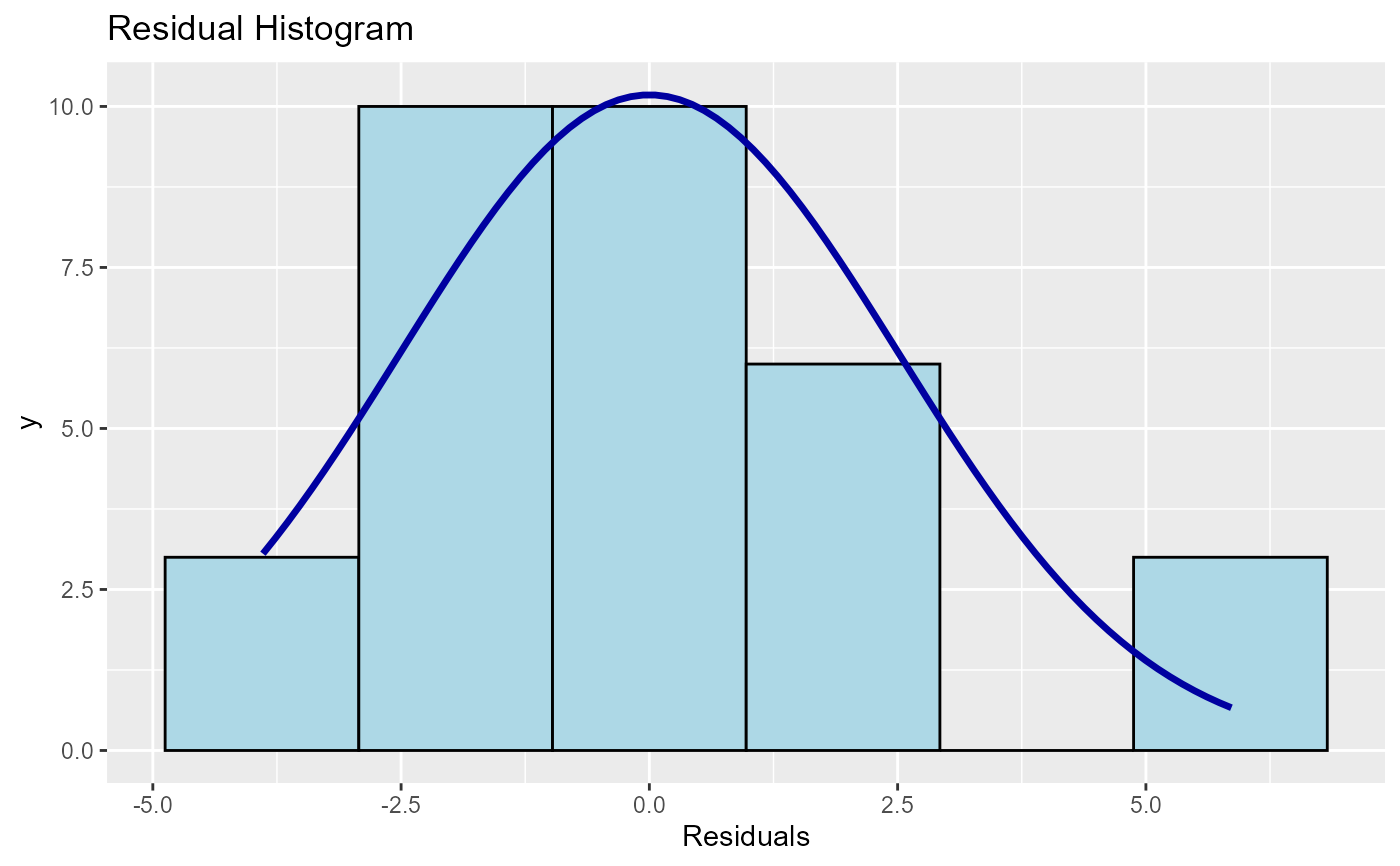Histogram of residuals for detecting violation of normality assumption.

ols_plot_resid_hist(model, print_plot = TRUE)

## Arguments

model An object of class lm. logical; if TRUE, prints the plot else returns a plot object.

## Deprecated Function

ols_rsd_hist() has been deprecated. Instead use ols_plot_resid_hist().

Other residual diagnostics: ols_plot_resid_box(), ols_plot_resid_fit(), ols_plot_resid_qq(), ols_test_correlation(), ols_test_normality()
model <- lm(mpg ~ disp + hp + wt, data = mtcars)
ols_plot_resid_hist(model)